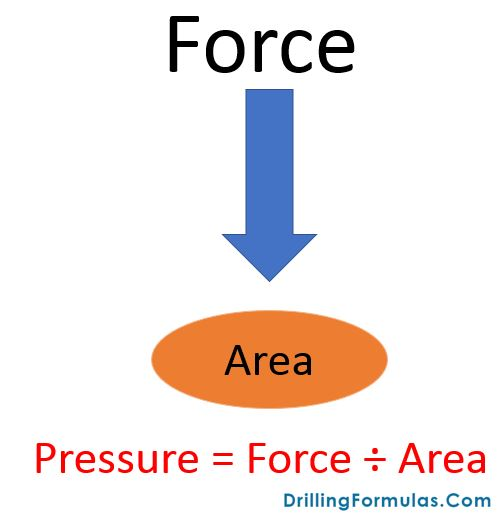# Pressure and Force Relationship and Its Application

Relationship of pressure, force and cross sectional area is one of the most commonly used concepts in oilfield and we will review this concept and its application. Pressure is force divided by cross section area (see an image below).Pressure = Force ÷ Area

We normally use pressure in many units such as psi (pound per square inch), Pascal, kg/m3, etc.

In drilling operation, we mostly use circular area so area can be calculated by this formula;

## Area = π x (radius)2 or π x (diameter)2÷ 4

Where  π= 22/7 = 3.143, so we can write a formula above in easy way

## Area = 3.143 x (radius)2 or 0.7857 x (diameter)2

Pressure calculation based on the relationship above is shown below;

## Pressure = force ÷ (3.143 x (radius)2) or force ÷ (0.7857 x (diameter)2)

Example : For this example, we will use the oilfield unit so force is in lb, diameter is in square inch (in2), and diameter is in inch.

Let’s try to apply pressure and force relationship in drilling operation. We plan to bullhead well and we still have drill string in the hole.

Drill string weight in the air = 45,000 lb
Mud weight in hole = 12.0 ppg
Bit size = 8.5”
Drill pipe size = 5″

What is the maximum pressure at surface you can apply before drilling string will be hydraulically pushed out due to bull heading pressure?

Solution

Buoyancy factor = (65.5 – 12.0) ÷ 65.5 = 0.817

Buoyed weight of drill string = 45,000 x 0.817 = 36,765 lb

Area = 0.7857 x (diameter)2= 0.7857 x (8.5)2= 56.77 square inch

Pressure = 36,765 lb ÷ 56.77 square inch= 647 psi.

In order to perform safe bullheading operation with drill string in hole, you need to apply bullheading pressure less than 647 psi on surface.Ref books: Lapeyrouse, N.J., 2002. Formulas and calculations for drilling, production and workover, Boston: Gulf Professional publishing.

Bourgoyne, A.J.T., Chenevert , M.E. & Millheim, K.K., 1986. SPE Textbook Series, Volume 2: Applied Drilling Engineering, Society of Petroleum Engineers.

Mitchell, R.F., Miska, S. & Aadny, B.S., 2011. Fundamentals of drilling engineering, Richardson, TX: Society of Petroleum Engineers.

Share the joyWorking in the oil field and loving to share knowledge.

### 5 Responses to Pressure and Force Relationship and Its Application

1.販売中 ステンカラーコート アウトレット says:

It’s very effortless to find out any topic on net as compared to textbooks, as I found this piece of writing at
this site.

2.jilani says:

Good information about pressure & Force

This site uses Akismet to reduce spam. Learn how your comment data is processed.